2017-12-03 17:28:28 arryCC 阅读数 3308
• ###### MATLAB图像处理

全面系统的学习MATLAB在图像处理中的应用

19860 人正在学习 去看看 魏伟

# 数字图像处理

## 一、学习内容总结

### 1. 第一章 绪论

1. 定义我们称之为数字图像处理领域的范围；
2. 通过考察几个领域，给出图像处理技术状况的概念；
3. 讨论图像处理用到的几种方法；
4. 概述通用目的的典型图像处理系统的组成。

#### 1.1 什么是数字图像处理

• 强度灰度：一幅图像可以被定义为一个二维函数 $f\left(x,y\right)$$f(x,y)$，其中 $x,y$$x,y$ 是空间（平面）坐标，而在任何一处的幅值 $f$$f$ 被称为在该点的灰度或强度。
• 数字图像：当 $x,y$$x,y$ 或灰度值 $f$$f$有限的离散数值时，称该图像为数字图像。也就是说数字图像是由有限数量的元素组成，每个元素都有特定的位置和幅值。这些元素被称为图画元素图像元素像素
• 数字图像处理 : 指用特定的计算机来处理数字图像。

#### 1.2 使用数字图像处理领域的实例

• 伽马射线成像：医学和天文。
• X射线成像：最早用于成像的电磁辐射源之一，医学诊断。
• 紫外波段成像 ：荧光显微镜。
• 可见光及红外线成像 ：可见显微镜技术，遥感，天气预测和预报，红外卫星图像，自动视觉检测，检测丢失的部件，指纹图像。
• 微波波段成像 ：雷达。
• 无线电波段成像 ：天文学和医学（核磁共振）。
• 其他方式 ：声波成像，电子显微镜方法，（由计算机产生的）合成图像。

#### 1.3 数字图像处理的基本步骤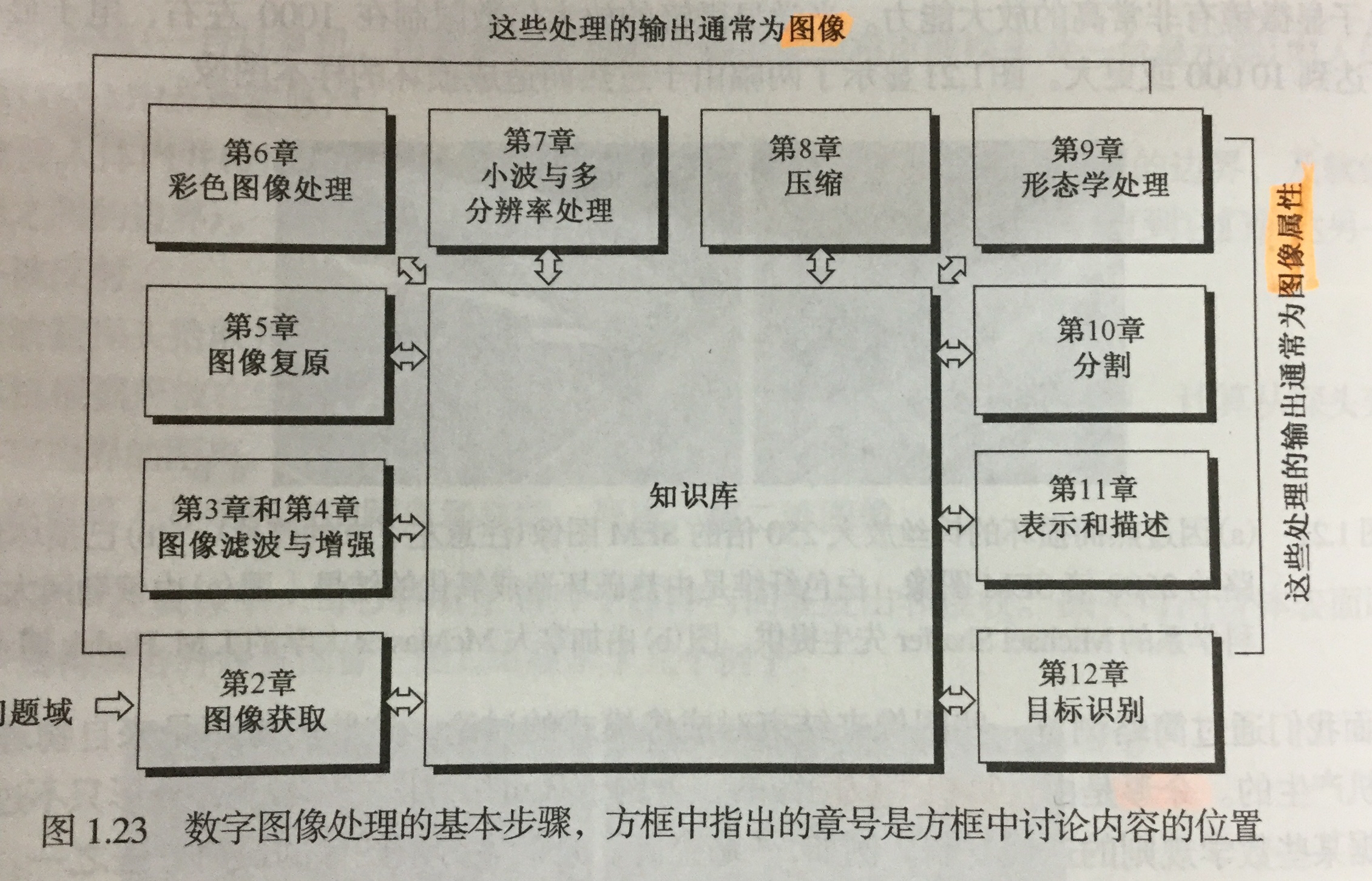#### 1.4 图像处理系统的组成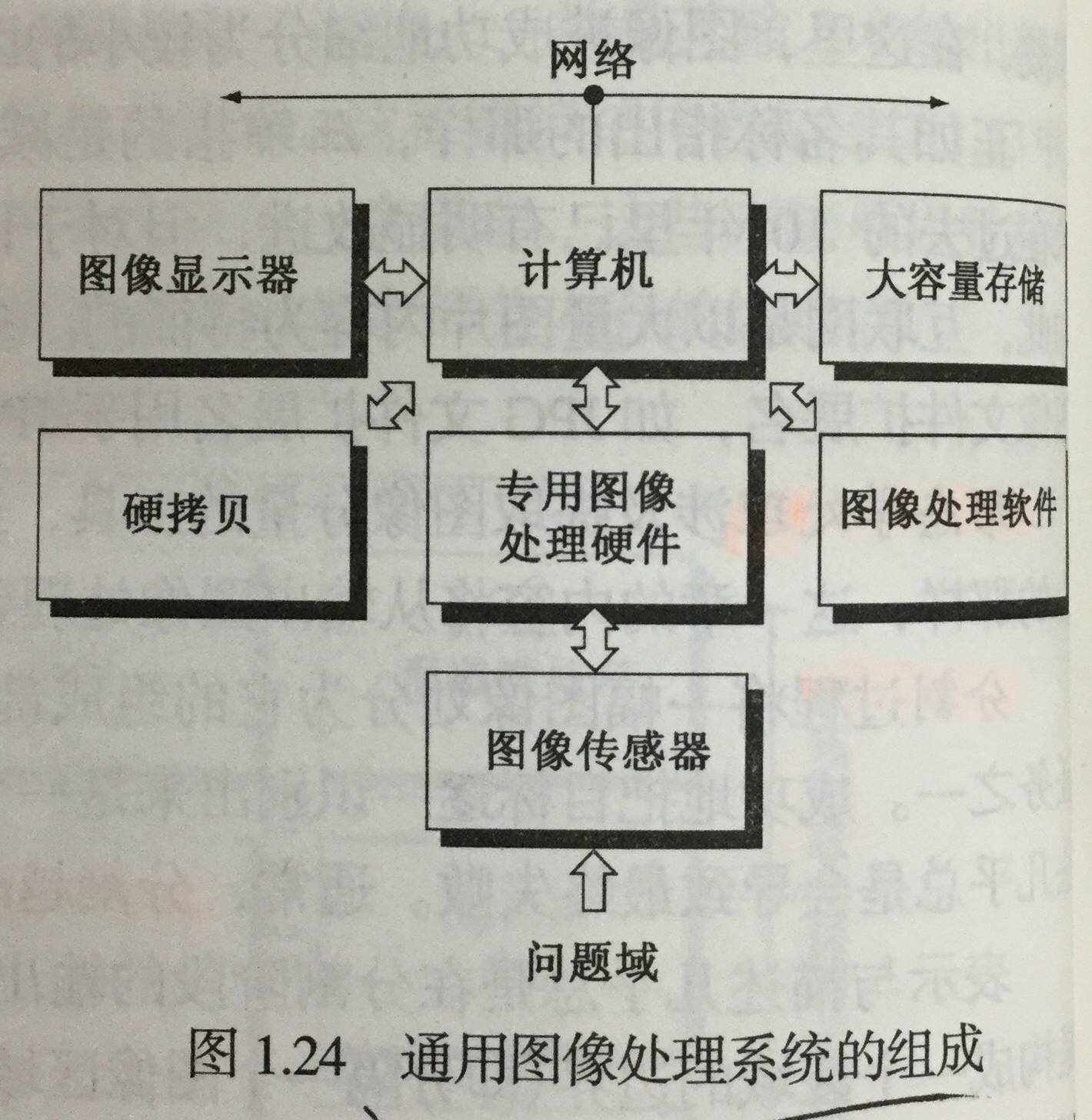### 2. 第二章 数字图像处理基础

#### 2.1 视觉感知要素

• 人眼的结构

重点介绍视网膜里的两类光感受器

• 锥状体 ：对颜色高度敏感，这种视觉称为白昼视觉或者亮视觉。高照明水平下执行。
• 杆状体 ：没有彩色感觉，对低照明度敏感，称为暗视觉或微光视觉。低照明水平下执行。
• 亮度适应与辨别

• 亮度适应现象 ：视觉系统不能同时在一个范围内工作，它是通过改变其整个灵敏度来完成这一较大变动的。
• 韦伯比 ：较大：亮度辨别能力较差；反之，较好。
• 感知亮度 不是简单的强度的函数

• 视觉系统往往会在不同强度区域的边界处出现“下冲”或“上冲”现象。
• 同时对比、错觉

#### 2.2 光和电磁波谱

• 电磁波是能量的一种，任何有能量的物体都会释放电磁波谱。它可以用 波长 ($\lambda$$\lambda$)、频率($v$$v$)或能量($E$$E$) 来描述，其中

$\lambda =c/v$

$E=hv$

• 光是一种特殊的电磁辐射，可以被人眼感知。
• 单色光 是没有颜色的光，也成为无色光。唯一属性就是它的强度或者大小，用 灰度级 来表示。单色图像常被称为 灰度图像
• 彩色光源 的质量可以用发光强度、光通量和亮度 来表示。

#### 2.3 图像感知和获取

• 图像获取方式

• 使用单个传感器来获取图像
• 使用条带传感器获取图像
• 使用传感器阵列获取图像
• 简单的图像形成模型

用形如 $f\left(x,y\right)$$f(x,y)$ 的二维函数来表示图像，那么：

$0

$f\left(x,y\right)$$f(x,y)$ 可以用两个分量来表征：

• 入射分量 入射到被观察场景的光源照射总量，用$i\left(x,y\right)$$i(x,y)$ 表示;
• 反射分量 场景中物体所反射的光照总量，用$r\left(x,y\right)$$r(x,y)$ 表示。

所以有：

$f\left(x,y\right)=i\left(x,y\right)r\left(x,y\right),0

#### 2.4 图像取样和量化

• 取样和量化的基本概念

• 取样 ：对坐标值进行数字化
• 量化 ： 对幅值数字化

数字图像的质量在很大程度上取决于取样和量化中所用的样本数灰度级

• 数字图像表示

用数列矩阵来表示一幅数字图像。在实数矩阵中，每个元素称为图像单元、图像元素或像素。

对比度 一幅图像最高和最低灰度级间的灰度差为对比度。

存储数字图像所用的比特数为：

$b=M×N×k,当M=N时，b={N}^{2}k$

灰度级数$L={2}^{k}$$L = 2^k$

• 空间和灰度分辨率

• 空间分辨率 ：图像中可辨别的最小细节的度量。在数量上，表示每单位距离线对数和每单位距离点数是最通用的度量（必须针对空间单位来规定才有意义）。
• 灰度分辨率 ：指在灰度级中可分辨的最小变化。
• 图像内插

用已知数据来估计未知位置的数据处理。是基本的图像重取样方法。可以处理图像的放大和缩小。

#### 2.5 像素间的基本关系

• 相邻像素

位于坐标 $\left(x,y\right)$$(x,y)$ 处的像素 $p$$p$ 有4个水平和垂直上的相邻像素，用 ${N}_{4}\left(p\right)$$N_4(p)$ 表示；有四个对角相邻像素，用 ${N}_{D}\left(p\right)$$N_D(p)$ 表示。如果 $p$$p$ 位于图像边界，则某些邻点可能 落在图像外边。

• 邻接性、连通性、区域和边界

• 4邻接、8邻接、混合邻接
• 距离度量

• 欧氏距离(圆)
• ${D}_{4}$$D_4$ 城市街区距离(菱形)
• 棋盘距离(正方形)

#### 2.6 常用数学工具介绍

• 阵列和矩阵操作
• 线性操作和非线性操作
• 算术操作
• 集合和逻辑操作
• 基本集合操作
• 逻辑操作
• 模糊集合
• 空间操作
• 单像素操作
• 邻域操作
• 几何空间变换与图像配准
• 向量和矩阵操作
• 图像变换

### 3.第三章

#### 3.1 背景知识

• 空间域 就是简单的包含图像像素的平面。空间域处理可用以下方式表示：

$g\left(x,y\right)=T\left[f\left(x,y\right)\right],T是在点\left(x,y\right)的邻域上处定义的一种算子$

• 灰度变换函数
$s=T\left(r\right),r,s分别代表处理前后的像素值$

#### 3.2 基本灰度变换函数

• 图像反转

得到灰度范围为 $\left[0,L-1\right]$$[0,L-1]$ 的一幅图像的反转图像:（得到等效的照片底片）

$s=L-1-r$

• 对数变换

对数变换的通用形式：

$s=clog\left(1+r\right)$

扩展图像中暗像素的值，同时压缩更高灰度级的值。反对数变换的作用与此相反。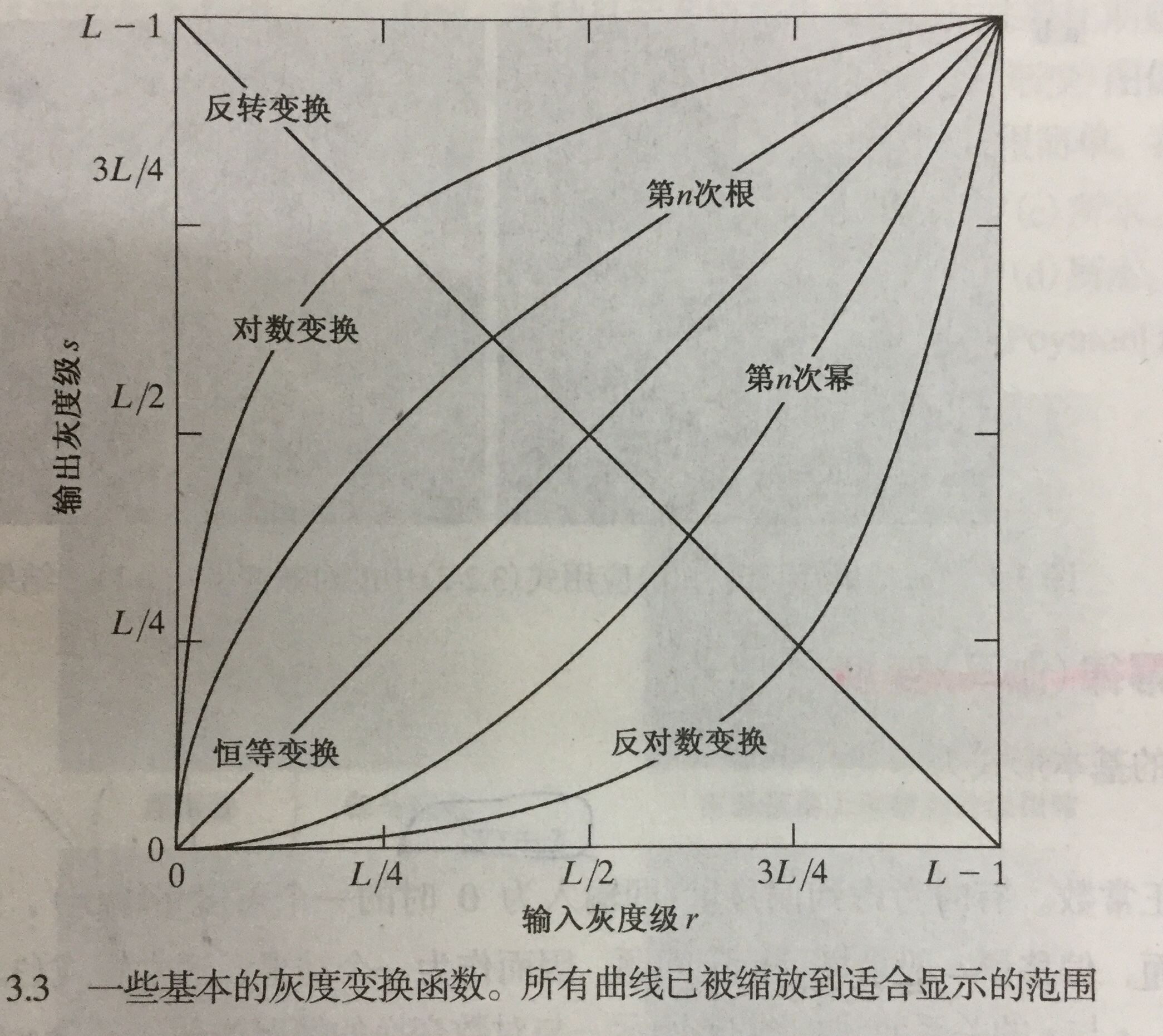• 幂律变换(伽马)变换

基本形式：

$s=c{r}^{\gamma }$

$\gamma <1$$\gamma < 1$ 变亮，大于1变暗，$c=\gamma =1$$c = \gamma = 1$ 恒等变换。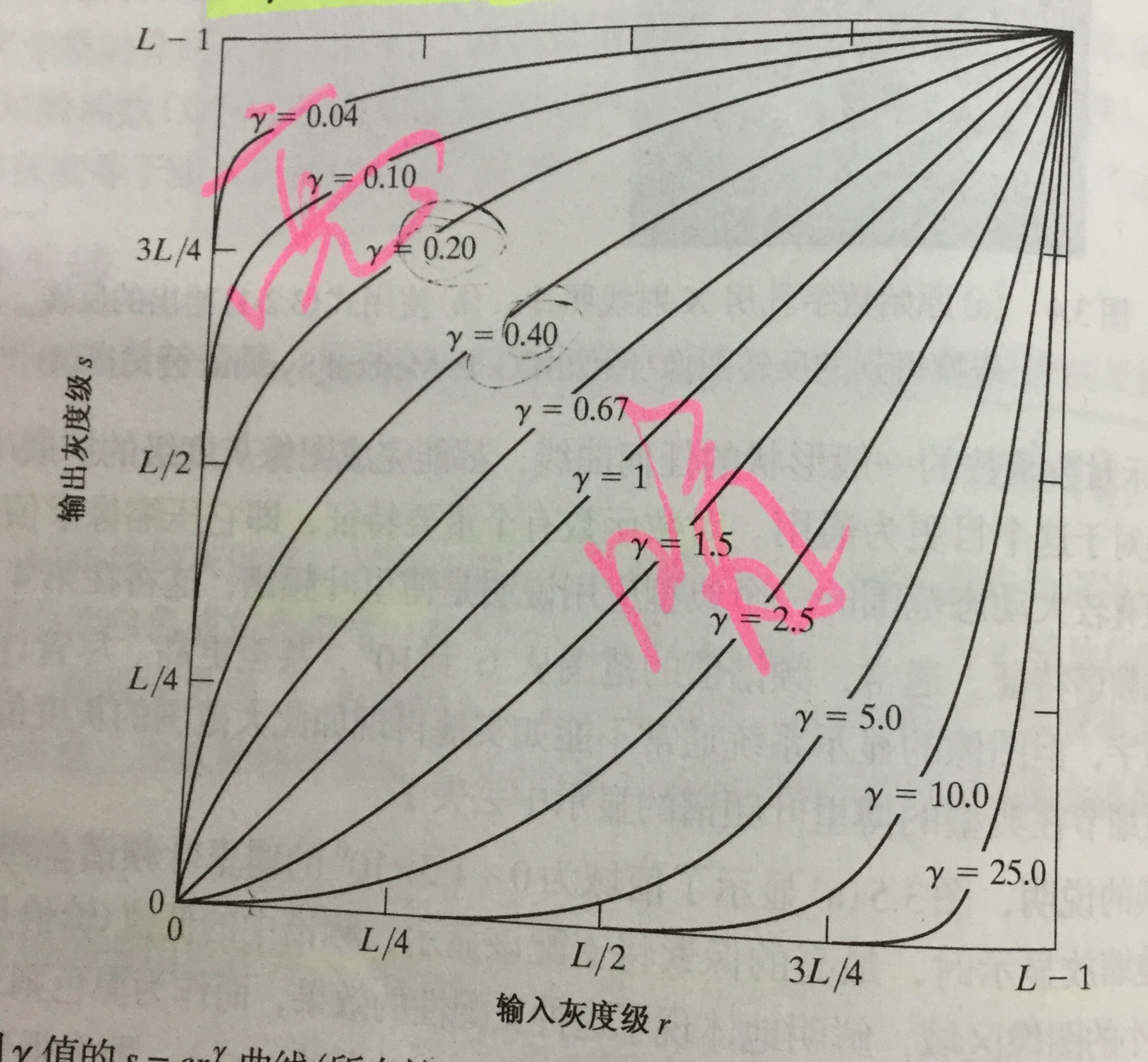* 分段线性变换函数
* 对比度拉伸：扩展图像灰度级动态范围处理，因此它可以跨越记录介质和显示装置的全部灰度范围。

根据$r,s$ 的取值，变换可以为线性函数和阈值处理函数。

• 灰度级分层：突出特定图像灰度范围的亮度。有两种方法：

• 突出范围 $\left[A,B\right]$$[A,B]$ 内的灰度，并将所有其他灰度降低到一个更低的级别；
• 突出范围$\left[A,N\right]$$[A,N]$ 内的灰度，并保持所有其他灰度级不变。
• 比特平面分层：突出特定比特为整个图像外观作贡献。

• 4个高阶比特平面，特别是最后两个比特平面，包含了在视觉上很重要的大多数数据。
• 低阶比特平面在图像中贡献更精细的灰度细节。

得出结论：储存四个高阶比特平面将允许我们以可接受的细节来重建原图像。这样可减少50%的存储量。

#### 3.3 直方图的处理

• 理论基础：若一幅图像的像素倾向于占据可能的灰度级并且分布均匀，则该图像会有高对比度的外观并展示灰色调的较大变化。

• 直方图均衡：

• 灰度范围为 $\left[0,L-1\right]$$[0,L-1]$ 的数字图像的直方图是离散函数 $h\left({r}_{k}\right)={n}_{k}$$h(r_k) = n_k$,其中 ${r}_{k}$$r_k$ 是第 $k$$k$ 级灰度值，${n}_{k}$$n_k$ 是图像中灰度为${r}_{k}$$r_k$ 的像素的个数。
• 通过转换函数$T\left({r}_{k}\right)$$T(r_k)$变换，得到直方图均衡化。
• 应用：自适应对比度增强。
• 直方图匹配：用于处理后有特殊直方图的方法。

• 局部直方图处理：以图像中每个像素邻域中的灰度分布为基础设计变换函数，来增强图像中小区域的细节。

• 在图像增强中使用直方图统计：提供这样一种增强图像的方法：

在仅处理均值和方差时，实际上直接从取样值来估计它们，不必计算直方图。这些估计被称为取样均值和取样方差。

#### 3.4 空间滤波基础

• 空间滤波机理

• 空间滤波器的组成：
• 一个邻域
• 对该邻域包围的图像像素执行的预定义操作

滤波产生的是一个新像素，新像素的坐标等于邻域中心的坐标，像素的值是滤波操作的结果。

• 空间相关与卷积

• 相关：滤波器模板移过图像并计算每个位置乘积之和的处理。一个大小为$m×n$$m×n$ 的滤波器与一幅图像 $f\left(x,y\right)$$f(x,y)$ 做相关操作，可表示为$w\left(x,y\right)☆f\left(x,y\right)$$w(x,y)☆f(x,y)$
• 卷积：与相关机理相似，但滤波器首先要旋转${180}^{o}$$180^o$ 一个大小为$m×n$$m×n$ 的滤波器与一幅图像 $f\left(x,y\right)$$f(x,y)$ 做j卷积操作，可表示为$w\left(x,y\right)★f\left(x,y\right)$$w(x,y)★f(x,y)$

#### 3.5 平滑空间滤波器

• 平滑线性滤波器(均值滤波器)

它使用滤波器确定的邻域内像素的平均灰度值代替图像中每个像素的值。应用：

• 降低噪声
• 灰度级数量不足而引起的伪轮廓效应的平滑处理
• 去除图像的不相关细节
• 统计排序(非线性)滤波器

最有代表性的是中值滤波器 ，特点：

• 将像素邻域内灰度的中值(在中值计算中，包括原像素值)代替该像素的值；
• 对处理脉冲噪声(椒盐噪声)非常有效。

#### 3.6 锐化空间滤波器

• 拉普拉斯算子：最简单的各向同性微分算子，是一个线性算子。因其为微分算子，因此强调的是图像中灰度的 突变而不是灰度级缓慢变换的区域。

• 非锐化隐蔽和高提升滤波：从原图像中减去一部分非锐化的版本。步骤：

• 模糊原图像
• 从原图像减去模糊图像
• 将模板加到原图像上
• 梯度：图像处理中的一阶微分用梯度实现。对于函数$f\left(x\right)$$f(x)$ ，在坐标$\left(x,y\right)$$(x,y)$ 处的梯度定义为二维列向量。它指出在位置$f\left(x,y\right)$$f(x,y)$$f$$f$的最大变化率方向。

应用：边缘增强。

### 4.第四章

#### 4.1. 基本概念

• 傅里叶概念：任何周期函数都可以表示为不同频率的正弦和或余弦和的形式，每个正弦项和或余弦项乘以不同的系数（傅里叶级数）。
• 傅里叶变换：在非周期函数用正弦和或余弦和乘以加权函数的积分来表示的公式。
• 介绍复数、傅里叶级数、冲击及其取样特征、连续函数的傅里叶变换以及之前提过的卷积。

#### 4.2. 取样与取样函数中的傅里叶变换

• 取样

在连续函数$f\left(x,y\right)$$f(x,y)$ 中模拟取样的一种方法是：用一个$\mathrm{\Delta }T$$\Delta T$ 单位间隔的冲击串作为取样函数去乘以$f\left(t\right)$$f(t)$ .

• 取样函数的傅里叶变换

空间域来两个函数乘积的傅里叶变换是两个函数在频率域的卷积。

• 取样定理

如果以超过函数最高频率的两倍的取样来获取样本，连续的带限函数可以完全从它的样本集来恢复。

#### 4.3. DFT小结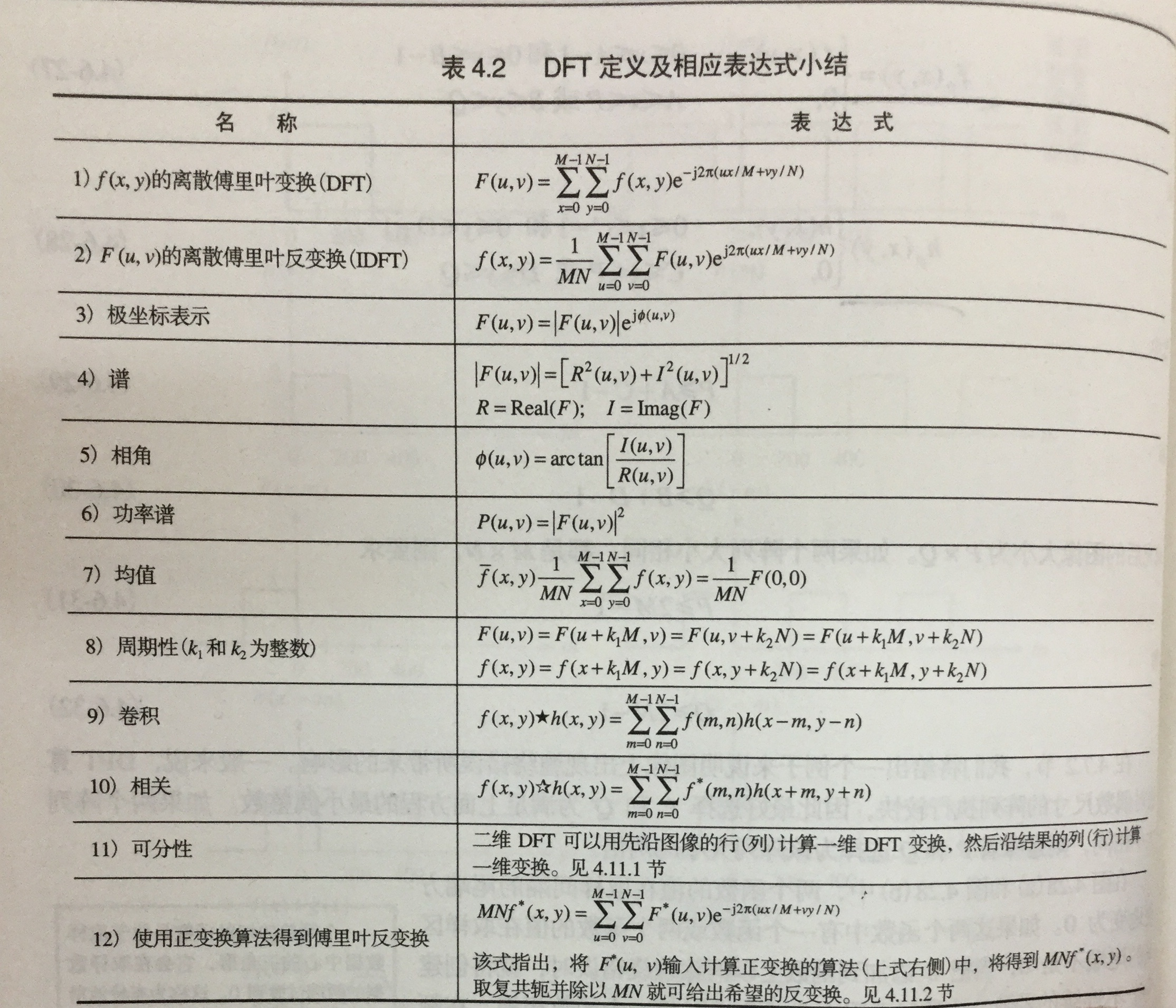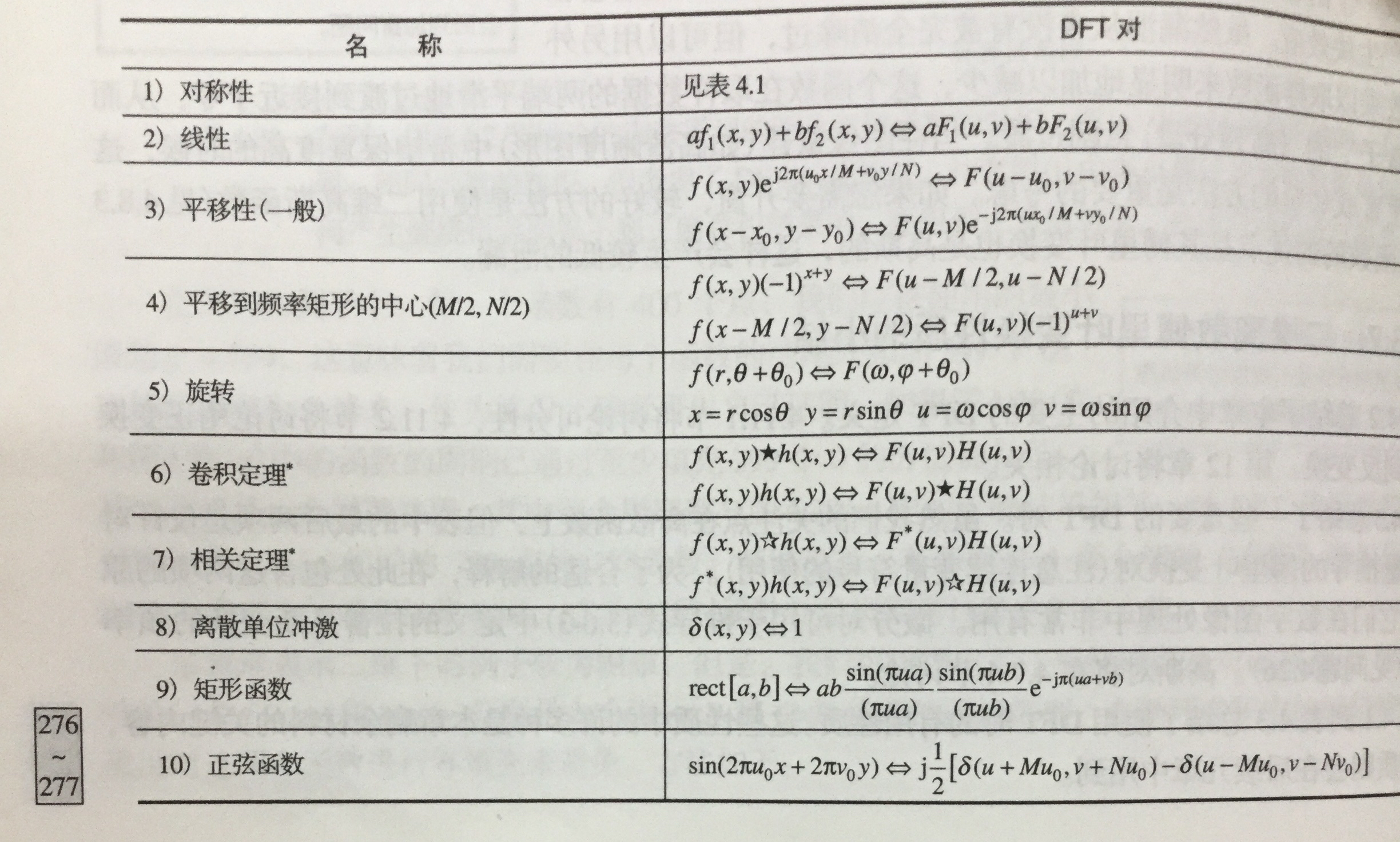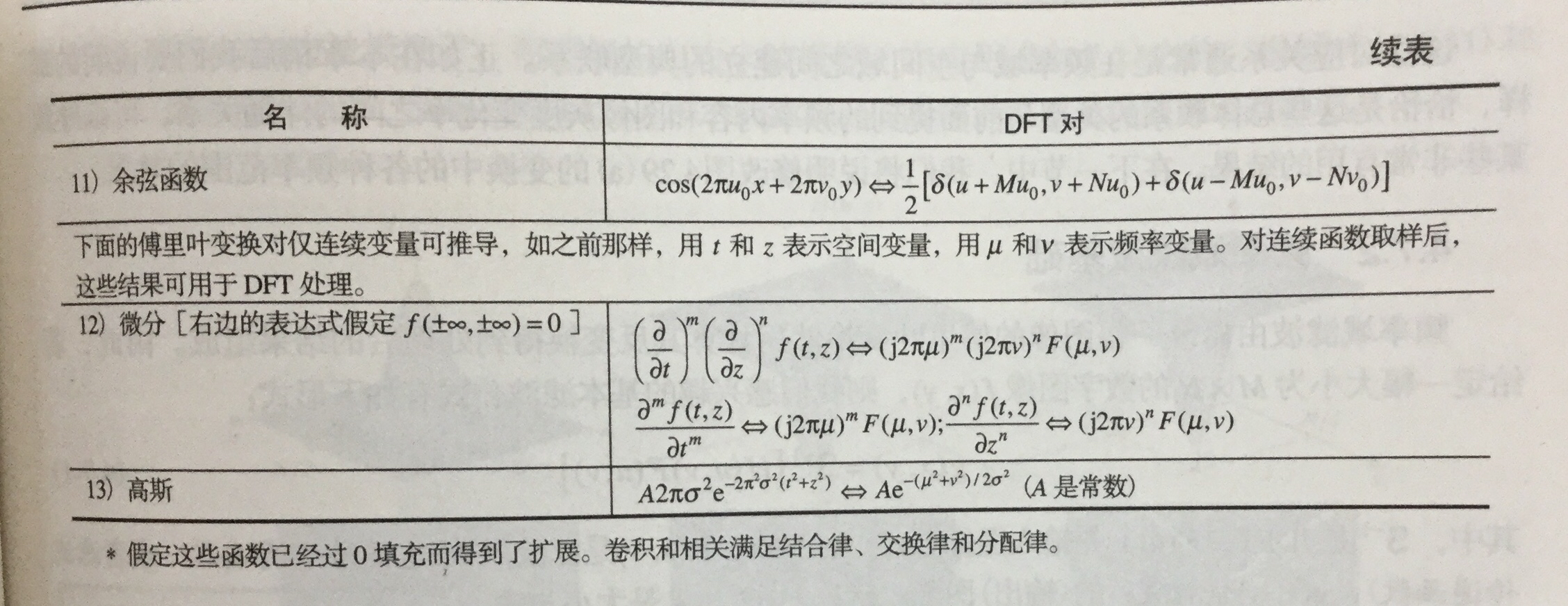#### 4.4. 频率域滤波

• 步骤
• 等到填充参数$P$$P$$Q$$Q$
• 形成大小为$P×Q$$P × Q$ 的填充后的图像${f}_{p}\left(x,y\right)$$f_p(x,y)$
• $\left(-1{\right)}^{x+y}$$(-1)^{x+y}$ 乘以${f}_{p}\left(x,y\right)$$f_p(x,y)$移到其变换中心
• 计算上一步骤的DTF，得到$F\left(u,v\right)$$F(u,v)$
• 生成实的、对称的滤波函数$H\left(u,v\right)$$H(u,v)$
• 得到处理后的图像${g}_{p}\left(x,y\right)$$g_p(x,y)$
• ${g}_{p}\left(x,y\right)$$g_p(x,y)$ 的做上限提取$M×N$$M×N$区域 ，得到最终的处理结果$g\left(x,y\right)$$g(x,y)$
• 空间域与频率域间的纽带是卷积定理。

#### 4.5. 使用频率域滤波器平滑图像

• 定义总结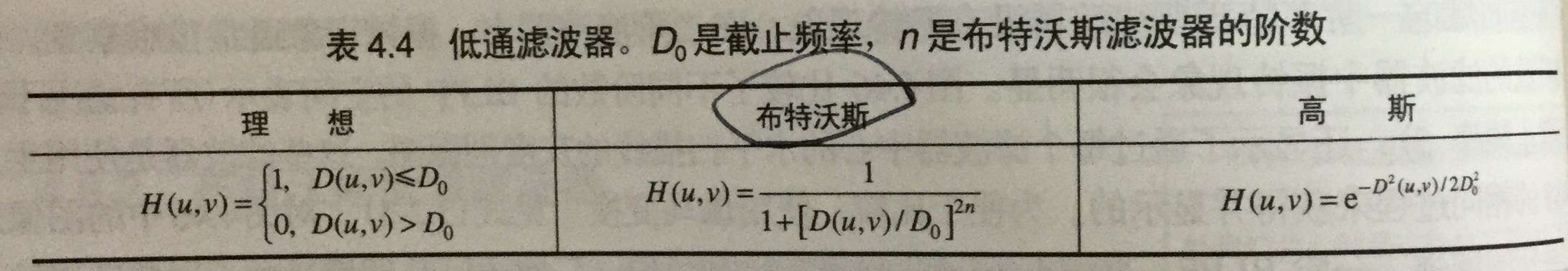• 特性
• 理想低通滤波器(ILFP)
• 特性：模糊和振铃。
• 布特沃斯低通滤波器(BLPF)
• 特性：随着阶数增高，其振铃和负值变明显。(一阶时无)
• 高斯低通滤波器(GLPF)
• 特性：无振铃

#### 4.6. 使用频率域滤波器锐化图像

• 三种高通滤波器来锐化图像
• 定义总结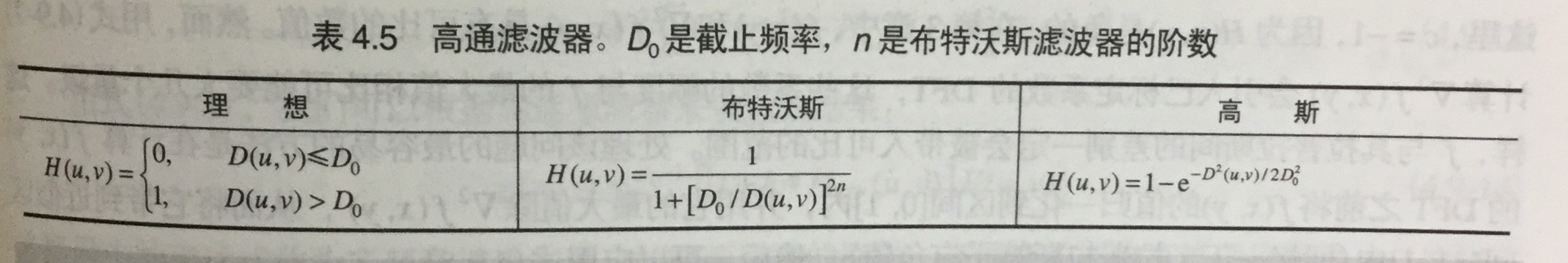* 特性

* 理想高通滤波器(IHPF)
* 有振铃
* 布特沃斯高通滤波器(BHPF)
* 比IHPF更平滑
* 高斯低通滤波器(GHPF)
* 比前两个更平滑，即使微小物体和细线条得到的结果也比较其清晰

• 其他方式

• 拉普拉斯算子
• 钝化模板、高提升滤波和高频强调滤波
• 同态滤波

#### 4.7.选择性滤波器

• 带阻滤波器和带通滤波器

• 带阻滤波器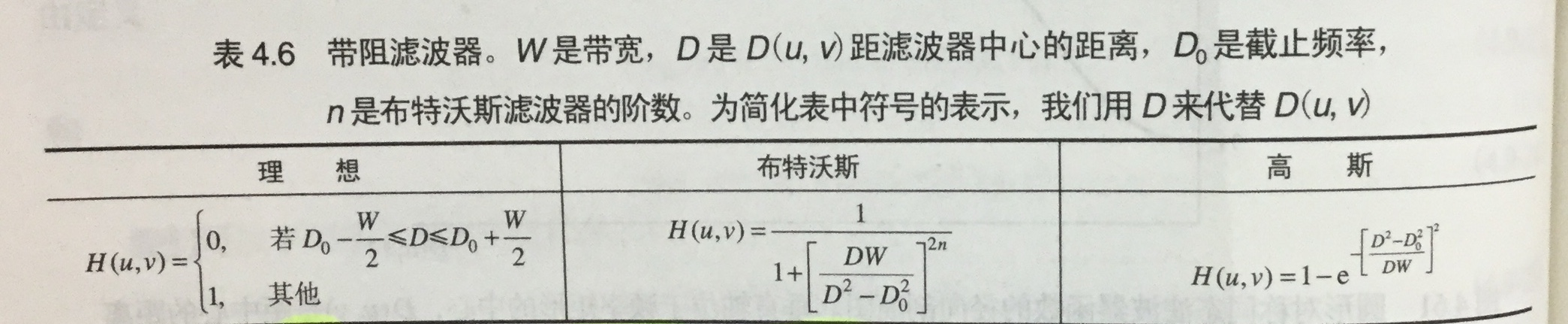• 带通滤波器

• 通过1减去带阻得到。

• 陷波滤波器：拒绝事先定义的关于频率矩形中心的一个邻域的频率。

• 陷波带阻滤波器

用中心已被平移到陷波滤波中心的高通滤波器的乘积来构造。

• 陷波带通滤波器

通过1减去带阻得到。

2017-03-19 18:50:27 Elaine1998 阅读数 2912
• ###### MATLAB图像处理

全面系统的学习MATLAB在图像处理中的应用

19860 人正在学习 去看看 魏伟

1.什么是数字图像？

2.数字图像的特点
①目前，数字图像处理的信息大多是二维信息，处理信息量很大。
②数字图像处理占用的频带较宽。
③数字图像中各个像素是不独立的，其相关性大。

3.什么是像素？

4.什么是分辨率？

5.什么是深度？

6.照片的大小怎么计算？

7.什么是采样？

8.什么是灰度？

9.几种常见的数字图像类型
①黑白图像：图像的每个像素只能是黑或白，没有中间的过渡，故又称二值图像；
②灰度图像：指每个像素的信息由一个量化的灰度级来描述的图像，没有彩色信息；
③彩色图像：指每个像素的信息由RGB三原色构成的图像，其中RGB是有不同的灰度级来描述的。2006-10-09 15:40:00 EmilMatthew 阅读数 6671
• ###### MATLAB图像处理

全面系统的学习MATLAB在图像处理中的应用

19860 人正在学习 去看看 魏伟

数字图像处理实践---夜间图像增强

EmilMatthew (EmilMatthew@126.com)

[  类别  ]算法创新、算法对比

[推荐指数]★★★★

[  摘要  ]本文提出了两种针对夜间灰度图像的增强算法：一种基于极暗区域连通集的分割算法，结合非线性动态调整及直方图均衡化方法对夜间图像进行增强，该算法的主要特点是对场景中的主要景物有较为突出的表现，轮廓清晰。

[ 关键词 ] 夜间视图图像增强、非线性调整、连通集、广度优先搜索、绿色通道

Digit Image Process Practice ------Night Image Enhancement

[Classify] Algorithm Innovation , Algorithm Comparison

[  Level ] ★★★★

[Abstract] This article introduces two methods to enhance the night pictures.  One method is based on the deeply dark area connected set partition algorithm , and both with the nonlinear adjusting method and equalized method. This algorithm has its special points such as enhancing the important part of the picture and has a clearly outline. Another method is based on the night picture’s grey histogram’s special property , changes its green channel to grey picture. This algorithm has its special points such as showing out the details vividly and has a good vision effect.

[Key Words] Night Vision , Image Enhancement , Nonlinear Dynamic Adjust Connected Set , Breadth First Search, Green Channel

[0引言]

夜间图像的主要特点是微光，暗色区域占据了画面的主要部分，相应的灰度直方图也集中分布于坐标系的左侧。因此，夜间图像的主要增强工作在于将较暗处的细节表达清楚，但又不能过亮，丢失过度的细节信息。提出了将对比度增强方法与直方图均衡算法级联的方法，虽然该方法本身会带来一定的噪声，但是该文的一个比较有益的启发在于将多种效果进行组合，以获得较好的处理效果。提出了一种针对BBHE（双直方均衡）算法改进的算法，该算法主要特点在于克服简并保持图像亮度进行增强。本文主要从图像的处理不同需求出发，考虑了两种不同的增强方案：方案一，基于极暗区域连通集的分割算法的处理目标更接进于让机器去“看”, 主要的特点是在于对于主要景物表现得十分清晰；而方案二，将绿色通道转成灰度图像，主要是针对灰度都集中分布在小于64的夜间灰度图像，处理结果更适合让人眼去看，主要特点在于体现画面的细节。

[1符号假设]

p: 最暗灰度像素的个数占总像素个数的百分比

[2基于极暗区域连通集的分割算法]

2.1想法的来源

该算法的想法是基于夜间图像的有较大的连通区域是暗区得出的，所谓暗区，是指某幅图像中最暗灰度像素的个数占总像素个数的一定百分比内(不妨设为p )的所有像素。下面是三幅测试图像及其灰度柱状图(注：由于个别像素所占比例过大，因此此处的灰度柱状图采取了“限高”处理)1：测试图像1及其灰度柱状图2：测试图像2及其灰度柱状图图3：测试图像3及其灰度柱状图

所以，即便都是在视觉上的暗图像，在灰度的分布上还是有所不同的，图1中灰度<5像素几乎可以忽略，而图2、图3灰度<5的像素则占到了全部像素的相当一部分比例。所以，划分最暗区域的原则不应以灰度的某个上限为限定，而应以某图中最暗灰度像素占所有像素的百分比p为依据。下面是三幅图像当p=0.15时提取的极暗区域情况：4：测试图像1（p=0.15）5：测试图像2（p=0.15）6：测试图像3（p=0.15）

通过对三幅测试图像极暗区域的提取，可以看出，极暗区域的连通特性在夜间图像是显然成立的。

2.1算法的核心部分

之所以要提出连通区域，主要是考虑，如果仅仅以p值作为极暗区域的划分，会将一些较暗，但是夹杂在较亮区域的像素作与连通的极暗区域相同的粗略处理，难以体现细节。

算法的整体框架如下：

1．  采用广度优先搜索，根据p值，找出极暗连通区域。

2．  对于非极暗连通区域的像素，作均值化处理。

1．  定义极暗连通区域，是以其像素值的个数点总像素的个数的百分比q

2．  之所以不再对极暗连通区域作均值化处理，主要是这样考虑的：因为即

算法的实现部分参附录

算法的实验结果：图7：测试图像1的算法效果对比图图8：测试图像2的算法效果对比图图9：测试图像3的算法效果对比图

从实验结果的对比中，可以看出：直方图均值化方法使得画面稍亮，而文献的方法除了会带来噪声外，亦在细节处使得画面有些模糊感，本文提出的“极暗连通区域分割+非极暗连通区域均值化”（简称DPEQU）方法，具有对比明显，重点突出的优点。

2.2算法的改进

此处的非线性动态范围调整的公式为：

g(i,j)=c·log(1+f(i,j)) (i=1,2,…,m j=1,2,…,n)

其中，c值的计算方式如下：

由于非线性动态范围调整对稍亮些的图像，如测试图像1，就会呈现调整过度的状况，因此，对这类图像（判定这类图像的条件为灰度像素级别<20的像素个数占不到全画面像素的15%），不做动态调整，而仅使用DPEQU

实验结果：从实验结果来看，较DPEQU算法的改进之处，在于画面的细节得到突出的体现，不失为一种针对夜间图像增强的有效方法。当然，这个方法的缺点也是存在的，那就是局部的亮区容易被调整的过亮，从面掩盖暗区的一些细节，因此，如何在增亮和突出细节上找到一个较好的“平衡点”，仍是一个值得思考和探索的问题。

[3绿色通道转成灰度图像的方法]

下面介绍的增强方法，可以说是有些讨巧的，虽然表面上看上去很简单，但是针对夜间图像大部分灰度像素值<64（即绿色通道表现力最强，而红、蓝两通道没有表现的区域中）的特性，所以将原图先进行灰度到绿色通道的转换，然后以绿色通道中的绿度像素级别直接以同级别的灰度像素进行输出，下面是三幅图像三个通道所对应的画面信息的展现：12：测试图像1三个通道信息13：测试图像2三个通道信息14：测试图像3三个通道信息

该算法的实现请参附录15：测试图像1经绿色通道转成灰度算法增强后的效果16：测试图像2经绿色通道转成灰度算法增强后的效果17：测试图像2经绿色通道转成灰度算法增强后的效果

从数学上看，该算法实际上是对于灰度像素<64的像素都做了乘以255/64的操作，对于灰度像素>64的像素点则简单处理成255。虽然是一个简单的线性变换，但是从结果上来看，在画面的真实感和细节表达上，该算法处理出的画面显然要胜过本文中所展示的其它增强效果，非常适合当用户的要求为人眼观察时使用。当然，本算法亦存在着一定的缺陷，那便是增强后的画面仍是偏暗的，如果使用相应的增强效果，如均衡化、非线性动态范围调整，又会回到将细节处的信息“吞噬”掉的老路上。所以，找到适合针对绿色通道转成的灰度图像进行增亮的算法，仍是一个值得探索和努力的方向。

[参考文献与网站]

 朱虹等著,数字图像处理基础，科学出版社，2005.

 张　宇、王希勤、彭应宁，一种用于夜间图像增强的算法，清华大学学报(自然科学版)，1999 年第39 卷第9 期 ,79～ 80.

 沈嘉励、张　宇、王秀坛，一种夜视图象处理的新算法，中国图象图形学报，2000 年6 月,第5 卷(A 版) 第6 期.

 马志峰、史彩成，自适应图像对比度模糊增强算法，激光与红外Vol. 36 , No. 3，2006年3月

 王国权、仲伟波，灰度图像增强算法的改进与实现研究，计算机应用研究，2004年第12期，p175-176.

 EmilMatthew, 玩玩数字图像处理

void BMParse::nightPhotoPationMethod

(HDC inHdc,int offsetX,int offsetY,float portionOfDark)

{

//0.Inner value declaration

int darkAreaPixelCount;

int darkAreaUpLimit;     //根据p值计算出的某幅图像灰度门限值

unsigned long totalPixelNum;

unsigned long darkAreaNum;

unsigned long tmpDarkAreaNum;

unsigned long parsedNum;

unsigned long totalDarkAreaNum;

unsigned long tmpULVal;

int histVal;

float histPercent;

int darkAreaPixelIndex;

int darkAreaSetNum;

int usefulDarkSetID; //有效的连通集的标号

int usefulSetNum;

ePoint tmpPoint,tmpPoint2;

PSingleRearSeqQueue searchQueue;

//valuable for equalize op

int    arrHists;

float  pArr;

long  totalHistVal;

unsigned long outputPixel;

int            tmpTableVal;

int i,j,k;

//1.Use Pation Method to indentify the very dark area

//1.0 determine the dark area up limit

searchQueue=createNullSingleRearSeqQueue();

darkAreaPixelCount=0;

darkAreaNum=0;

totalPixelNum=mBMFileInfo.bmHeight*mBMFileInfo.bmWidth;

for(i=0;i<256;i++)

{

histVal[i]=0;

histPercent[i]=0;

}

for(i=0;i<mBMFileInfo.bmHeight;i++)

for(j=0;j<mBMFileInfo.bmWidth;j++)

{

histVal[bmpBWMatrix[i][j]]++;

bmpBWBackMatrix[i][j]=0;

}

for(i=0;i<256;i++)

histPercent[i]=(float)histVal[i]/(float)totalPixelNum;

i=0;

while(darkAreaPixelCount<6&&i<256)

{

if(histPercent[i]>0)

{

darkAreaPixelIndex[darkAreaPixelCount]=i;

darkAreaPixelCount++;

darkAreaNum+=histVal[i];

}

i++;

}

//check if darkAreaPixel>=totalPixelNum*portionOfDark

if(darkAreaNum<totalPixelNum*portionOfDark)

{

while(darkAreaNum<totalPixelNum*portionOfDark)

{

if(histPercent[i]>0)

{

darkAreaPixelIndex[darkAreaPixelCount]=i;

darkAreaNum+=histVal[i];

darkAreaPixelCount++;

}

i++;

}

}

//exception consult

if(i==256)

{

return;

}

//1.1 find dark area set

darkAreaUpLimit=i-1;

darkAreaSetNum=0;

usefulSetNum=0;

parsedNum=0;

totalDarkAreaNum=0;

for(i=0;i<mBMFileInfo.bmHeight;i++)

for(j=0;j<mBMFileInfo.bmWidth;j++)

{

if(bmpBWBackMatrix[i][j]==0)

{

if(bmpBWMatrix[i][j]>darkAreaUpLimit)

{

parsedNum++;

bmpBWBackMatrix[i][j]=-1;//    marked ,not in dark area

}

else

{

//a.init part of the breadth first search

darkAreaSetNum++;

tmpDarkAreaNum=0;

tmpPoint.x=j;

tmpPoint.y=i;

enQueue(searchQueue,tmpPoint);

//b.core part of search

while(!isEmpty(searchQueue))

{

parsedNum++;

//save to count values                                                                              bmpBWBackMatrix[tmpPoint.y][tmpPoint.x]=darkAreaSetNum;

tmpDarkAreaNum++;

//left -> bmpBWMatrix[tmpPoint.y][tmpPoint.x-1]

if(tmpPoint.x>0)

{                                                                             if(bmpBWBackMatrix[tmpPoint.y][tmpPoint.x-1]==0&&

bmpBWBackMatrix[tmpPoint.y][tmpPoint.x-1]!=-3&&

bmpBWMatrix[tmpPoint.y][tmpPoint.x-1]<=darkAreaUpLimit)

{

tmpPoint2.x=tmpPoint.x-1;

tmpPoint2.y=tmpPoint.y;                                                                                   bmpBWBackMatrix[tmpPoint.y][tmpPoint.x-1]=-3;                                    enQueue(searchQueue,tmpPoint2);                                         }

}

//up  -> bmpBWMatrix[tmpPoint.y-1][tmpPoint.x]

if(tmpPoint.y>0)

{

if(bmpBWBackMatrix[tmpPoint.y-1][tmpPoint.x]==0&&

bmpBWBackMatrix[tmpPoint.y-1][tmpPoint.x]!=-3&&

bmpBWMatrix[tmpPoint.y-1][tmpPoint.x]<=darkAreaUpLimit)

{

tmpPoint2.x=tmpPoint.x;                                                                                   tmpPoint2.y=tmpPoint.y-1;                                                                               bmpBWBackMatrix[tmpPoint.y-1][tmpPoint.x]=-3;

enQueue(searchQueue,tmpPoint2);                                                            }

}

//right -> bmpBWMatrix[tmpPoint.y][tmpPoint.x+1]

if(tmpPoint.x<mBMFileInfo.bmWidth-1)

{

if(bmpBWBackMatrix[tmpPoint.y][tmpPoint.x+1]==0&&

bmpBWBackMatrix[tmpPoint.y][tmpPoint.x+1]!=-3&&

bmpBWMatrix[tmpPoint.y][tmpPoint.x+1]<=darkAreaUpLimit)

{                                                                                                        tmpPoint2.x=tmpPoint.x+1;

tmpPoint2.y=tmpPoint.y;

bmpBWBackMatrix[tmpPoint.y][tmpPoint.x+1]=-3;

enQueue(searchQueue,tmpPoint2);

}

}

//down -> bmpBWMatrix[tmpPoint.y+1][tmpPoint.x]

if(tmpPoint.y<mBMFileInfo.bmHeight-1)

{                                                        if(bmpBWBackMatrix[tmpPoint.y+1][tmpPoint.x]==0&&

bmpBWBackMatrix[tmpPoint.y+1][tmpPoint.x]!=-3&&

bmpBWMatrix[tmpPoint.y+1][tmpPoint.x]<=darkAreaUpLimit)

{

tmpPoint2.x=tmpPoint.x;

tmpPoint2.y=tmpPoint.y+1;                                                                                  bmpBWBackMatrix[tmpPoint.y+1][tmpPoint.x]=-3;                                       enQueue(searchQueue,tmpPoint2);                                                      }

}

deQueue(searchQueue);

}

if(tmpDarkAreaNum>0.02*totalPixelNum)

{                                                              usefulDarkSetID[usefulSetNum]=darkAreaSetNum;

usefulSetNum++;

totalDarkAreaNum+=tmpDarkAreaNum;

}

}

}

}

for(i=0;i<mBMFileInfo.bmHeight;i++)

for(j=0;j<mBMFileInfo.bmWidth;j++)

{

if(bmpBWBackMatrix[i][j]>0)

{

for(k=0;k<usefulSetNum;k++)

{

if(bmpBWBackMatrix[i][j]==usefulDarkSetID[k])

break;

}

if(k==usefulSetNum)

bmpBWBackMatrix[i][j]=-2;

else

bmpBWBackMatrix[i][j]=0;

}

}

for(i=0;i<mBMFileInfo.bmHeight;i++)

for(j=0;j<mBMFileInfo.bmWidth;j++)

if(bmpBWBackMatrix[i][j]!=0&&bmpBWBackMatrix[i][j]!=-1&&

bmpBWBackMatrix[i][j]!=-2)

{

return;

}

//2.Use equalize method to process the picture

//2.1 cal each grey value's fequence

for(i=0;i<256;i++)

arrHists[i]=0;

for(j=0;j<mBMFileInfo.bmHeight;j++)

for(i=0;i<mBMFileInfo.bmWidth;i++)

{                                          if(bmpBWBackMatrix[j][i]==-1||bmpBWBackMatrix[j][i]==-2)

arrHists[bmpBWMatrix[j][i]]++;

}

//2.cal total hist value

totalHistVal=0;

for(i=0;i<256;i++)

totalHistVal+=arrHists[i];

pArr=0;

pArr=(float)(arrHists+arrHists)/(float)(2*totalHistVal);

for(i=2;i<256;i++)

pArr[i]=pArr[i-1]+(float)arrHists[i]/(float)totalHistVal;

for(i=0;i<mBMFileInfo.bmHeight;i++)

for(j=0;j<mBMFileInfo.bmWidth;j++)

{                                  if(bmpBWBackMatrix[i][j]==-1||bmpBWBackMatrix[i][j]==-2)

{

tmpTableVal=255*pArr[bmpBWMatrix[i][j]];

}

else

tmpTableVal=bmpBWMatrix[i][j];

//make to windows color format

outputPixel=((unsigned long)paletteArr[tmpTableVal].b)*65536+

((unsigned long)paletteArr[tmpTableVal].g)*256+

(unsigned long)paletteArr[tmpTableVal].r;

//output pixel                        SetPixel(inHdc,j+offsetX,i+offsetY,outputPixel);

bmpBWBackMatrix[i][j]=tmpTableVal;

}

}

1 bmpBWBackMatrix是程序中的一个重要的结构，其值的具体意义如下：

-3：在搜索过程中被新吸收的可接纳点，0：未搜索，>0 ：属于已搜索区域。

-2：表示像素点属于极暗区域，但是所在区域不为连通集。

-1：表示像素点属于非极暗区域。

0 ：表示像素点属于极暗连通区域。

2 搜索连通集的过程采用队列实现了广度优先搜索。

{

//0. Valuables declaration:

float plusConstant;

float plusConstantChanged;

int maxGreyValue,minGreyValue,spanGreyValue;

int avgGreyVal;

int tmpTableVal;

unsigned long sumGreyVal;

unsigned long outputPixel;

unsigned long totalPixelNum;

unsigned long darkAreaNum;

int i,j;

darkAreaNum=0;

totalPixelNum=mBMFileInfo.bmHeight*mBMFileInfo.bmWidth;

for(i=0;i<mBMFileInfo.bmHeight;i++)

for(j=0;j<mBMFileInfo.bmWidth;j++)

{

if(bmpBWMatrix[i][j]<20)

darkAreaNum++;

}

if(darkAreaNum<0.15*totalPixelNum)

return;

//1.Core Process

//1.0Common Process

// find max and min greyvalue

maxGreyValue=-1;

minGreyValue=266;

for(i=0;i<mBMFileInfo.bmHeight;i++)

for(j=0;j<mBMFileInfo.bmWidth;j++)

{

if(bmpBWMatrix[i][j]>maxGreyValue)

{                                                                         maxGreyValue=bmpBWMatrix[i][j];

}

if(bmpBWMatrix[i][j]<minGreyValue)

{

minGreyValue=bmpBWMatrix[i][j];

}

}

//2.0.cal plus constant value

spanGreyValue=maxGreyValue-minGreyValue;

plusConstant=spanGreyValue/log10(1+spanGreyValue);

for(i=0;i<mBMFileInfo.bmHeight;i++)

for(j=0;j<mBMFileInfo.bmWidth;j++)

{

//cal adjusted grey table value index

tmpTableVal=(int)(plusConstant*log10(1+bmpBWMatrix[i][j]));

//copy to back bw matrix , for next step of processing use

bmpBWMatrix[i][j]=tmpTableVal;

}

}

void BMParse::changeGreenChannelToGrey

(HDC inHdc,int offsetX,int offsetY)

{

//0.Inner Value declaration

unsigned long outputPixel;

int tmpTableVal;

int i,j;

//1.Core part of false color change

for(i=0;i<mBMFileInfo.bmHeight;i++)

for(j=0;j<mBMFileInfo.bmWidth;j++)

{

//green channel

if(bmpBWMatrix[i][j]<64)                                                                                    tmpTableVal=255/64*bmpBWMatrix[i][j];

else if(bmpBWMatrix[i][j]<192)

tmpTableVal=255;

else                                                                                   tmpTableVal=-255/63*(bmpBWMatrix[i][j]-192)+255;

//make to windows color format

outputPixel=((unsigned long)paletteArr[tmpTableVal].b)*65536+

((unsigned long)paletteArr[tmpTableVal].g)*256+

(unsigned long)paletteArr[tmpTableVal].r;

//1.2show out

SetPixel(inHdc,j+offsetX,i+offsetY,outputPixel);

bmpBWBackMatrix[i][j]=tmpTableVal;

}

}

[源码下载、源程序下载]

Code1:直方图均衡化

Code2:文献中的方法实现

Code4:绿色通道转成灰度图像

[参考文献下载]

2019-12-20 10:36:34 rong11417 阅读数 139
• ###### MATLAB图像处理

全面系统的学习MATLAB在图像处理中的应用

19860 人正在学习 去看看 魏伟

1.介绍

2.模拟图像处理

3.数字图像处理

4.什么是图像

5.数字图像和信号之间的关系

6.如何形成数字图像

7.应用

## 4.什么是图像128 30 123 232 123 321 123 77 89 80 255 255

## 7.应用

### 机器/计算机视觉### 计算机图形学### 信号处理

2014-10-12 13:25:40 wozhendebuhaoma 阅读数 2759
• ###### MATLAB图像处理

全面系统的学习MATLAB在图像处理中的应用

19860 人正在学习 去看看 魏伟

http://zhidao.baidu.com/question/519528446090102645.html

http://zhidao.baidu.com/question/391593318895377005.html

http://zhidao.baidu.com/question/617082845639831412.html

http://zhidao.baidu.com/question/1946820750805557828.html

http://zhidao.baidu.com/question/1702286530212076180.html

http://zhidao.baidu.com/question/1238528588771891699.html

http://zhidao.baidu.com/question/1238528652585891459.html

http://zhidao.baidu.com/question/2010245200610808468.html

http://zhidao.baidu.com/question/2010308752180544028.html

http://zhidao.baidu.com/question/1238592204736973419.html

http://zhidao.baidu.com/question/2010309201259490868.html

http://zhidao.baidu.com/question/680698400065462692.html

http://zhidao.baidu.com/question/455208937458346285.html

http://zhidao.baidu.com/question/1860437354781339187.html

http://zhidao.baidu.com/question/1238656589677680619.html

http://zhidao.baidu.com/question/1238656653749463099.html

http://zhidao.baidu.com/question/680698721128169452.html

http://zhidao.baidu.com/question/1860437611195301507.html

http://zhidao.baidu.com/question/1860437674878353067.html